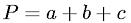Geometry > Length/PerimeterShowing results 1 to 6 of 6, on page 1 of 1
 DESCRIPTION EQUATION Arclength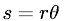Perimeter of a Circle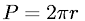Perimeter of a Rectangle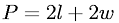Perimeter of a Regular Polygon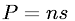Perimeter of a SquarePerimeter of a Triangle